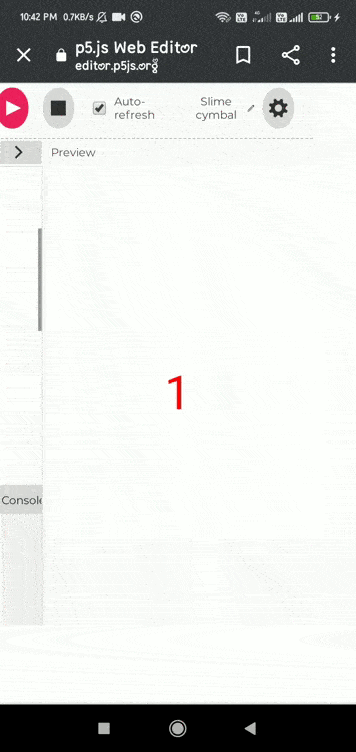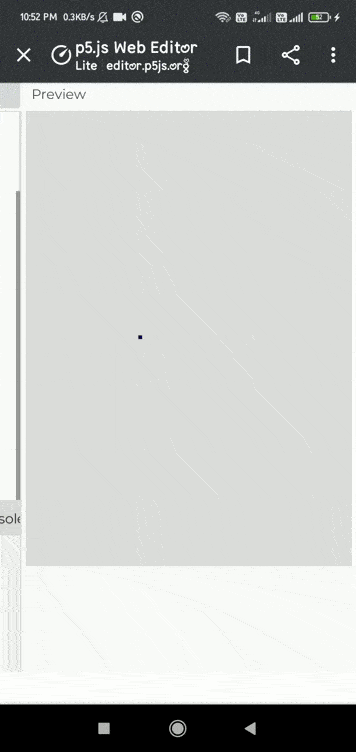# p5.js accelerationY variable

• Last Updated : 10 May, 2021

The accelerationY system variable is responsible for the acceleration of the device(tablets or mobile phones) along the y-axis. It can be used with the draw() function to accelerate the device in the y-axis of the coordinate system.

Its Value is represented as meters per second squared.

Syntax:

`accelerationY`

Example 1:

## Javascript

 `// Move a touchscreen device to register ` `// Acceleration changes. ` `function` `setup() ` `{ ` `  ``createCanvas(400,400) ` `} ` ` `  `function` `draw() { ` `  ``background(270); ` `   `  `  ``fill(``'red'``); ` `  ``textAlign(CENTER,CENTER); ` `  ``textSize(50); ` `  ``// Convert the acceleration into integer when ` `  ``// Device is moved along y-axis. ` `  ``text(int(accelerationY),windowWidth/2,windowHeight/2); ` `}`

Output:Example 2:

## Javascript

 `// Move a touchscreen device to register ` `// acceleration changes. ` `function` `draw() { ` `  ``createCanvas(400,400); ` `  ``background(220); ` `  ``fill(``'blue'``); ` `   `  `  ``//Set the variable. ` `  ``square(width/2, height/2, accelerationY); ` `}`

Output:My Personal Notes arrow_drop_up
Recommended Articles
Page :